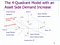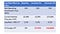# Non-linearity of Cap Rate Effects on Real Estate Price

Housing price is known to be strongly affected by the capitalization rate of real estate markets, and has been incorporated into the DW 4Q Model . However the non-linearity effects of cap rate on real estate price is not reflected accurately in the Model. (Figure 1)Figure 1 DW 4Q Model showing Cap Rate Effect on Property Price. Source: Geltner

Figure 1 shows that by rotating the line from the slope 11% to 8% on the second quadrant representing the change of cap rate, the relationship between rent and price is changed. However, it is more or less a linear effect. But the formula in fact implies a non-linear effect of cap rate on property price, as illustrated in the following example.Figure 1 Illustration of the non-linearity effect of Cap Rate on Price.

The example in Figure 1 shows that an increase and a decrease of 2% of cap rate would result in -47.62% and +10,000% change in property price, when the baseline cap rate is 2.2%. It clearly reflects the non-linearity effect. In contrast, if the baseline cap rate is 10%, then a 2% increase and decrease of cap rate would have a totally different effect on property price as shown in Figure 3.

When the baseline cap rate is 10% (as in the 1980s), an increase or a decrease of 2% of cap rate would only result in -16.67% and +25% change on property price. The effect is quite linear.

Figure 3 shows that when the cap rate drops from 4% to 3%, property price can increase by 33%. But when the cap rate drops from 2% to 1%, property price can increase by 100%. Furthermore, when the cap rate drops from 0.5% to 0.4%, property price can increase by 400%!

In fact, since the Global Financial Crisis in 2008, we have experienced an unprecedentedly long period of ultra-low interest rate, thus the effect of cap rate on property price becomes unprecedentedly great! When the same change of cap rate in the 1980s just caused property price change by 20%, but the same magnitude of change in the 2010s can cause property price change by 400%!

References

 DiPasquale, D. and Wheaton, W.C. (1992) The Markets for Real Estate Assets and Space: A Conceptual Framework, Journal of the American Real Estate and Urban Economics Association 20(1), 181–197. http://www00.unibg.it/dati/corsi/60002/37673-DiPasquale-Wheaton.pdf